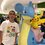# Formula for Root Product

In solving this problem, I found a formula for a product $(1+x_{1})(1+x_{2})(1+x_{3})(1+x_{4})...(1+x_{d})$ up until the last root of a polynomial of degree d. I challenge Brilliant members to find this formula, with proof.Note by Tristan Shin
7 years, 2 months ago

This discussion board is a place to discuss our Daily Challenges and the math and science related to those challenges. Explanations are more than just a solution — they should explain the steps and thinking strategies that you used to obtain the solution. Comments should further the discussion of math and science.

When posting on Brilliant:

• Use the emojis to react to an explanation, whether you're congratulating a job well done , or just really confused .
• Ask specific questions about the challenge or the steps in somebody's explanation. Well-posed questions can add a lot to the discussion, but posting "I don't understand!" doesn't help anyone.
• Try to contribute something new to the discussion, whether it is an extension, generalization or other idea related to the challenge.
• Stay on topic — we're all here to learn more about math and science, not to hear about your favorite get-rich-quick scheme or current world events.

MarkdownAppears as
*italics* or _italics_ italics
**bold** or __bold__ bold
- bulleted- list
• bulleted
• list
1. numbered2. list
1. numbered
2. list
Note: you must add a full line of space before and after lists for them to show up correctly
paragraph 1paragraph 2

paragraph 1

paragraph 2

[example link](https://brilliant.org)example link
> This is a quote
This is a quote
    # I indented these lines
# 4 spaces, and now they show
# up as a code block.

print "hello world"
# I indented these lines
# 4 spaces, and now they show
# up as a code block.

print "hello world"
MathAppears as
Remember to wrap math in $$ ... $$ or $ ... $ to ensure proper formatting.
2 \times 3 $2 \times 3$
2^{34} $2^{34}$
a_{i-1} $a_{i-1}$
\frac{2}{3} $\frac{2}{3}$
\sqrt{2} $\sqrt{2}$
\sum_{i=1}^3 $\sum_{i=1}^3$
\sin \theta $\sin \theta$
\boxed{123} $\boxed{123}$

## Comments

Sort by:

Top Newest

it would be kind enough if u tell us......

- 7 years, 2 months ago

Log in to reply

There is a pattern.....for example...............

( a + 1 ) ( b + 1 ) ( C + 1 ) = 1 + a + b + c + a b + b c + c a + a b c .

Hope you want this!

- 7 years, 2 months ago

Log in to reply

Yes, this is the pattern, but it can be simplified even further. Think about the certain terms in your example.

- 7 years, 2 months ago

Log in to reply

Well, Tristan, I can't get it......please post the answer.....

- 7 years, 2 months ago

Log in to reply

Let the polynomial be $f(x)$ with degree $n$ and roots $r_1, r_2, \dots, r_{n-1}, r_{n}$

Suppose $f(x) = a_0x^n + a_1x^{n-1} + \dots + a_{n-1}x + a_n = a_0(x-r_1)(x-r_2)\dots(x-r_{n-1})(x-r_{n})$

Then $f(-1) = a_0(-1)^n + a_1(-1)^{n-1} + \dots + a_{n-1}(-1) + a_n = a_0(-1-r_1)(-1-r_2)\dots(-1-r_{n-1})(-1-r_n)$

$f(-1) = a_0(-1)(1+r_1)(-1)(1+r_2)\dots(-1)(1+r_{n-1})(-1)(1+r_n)$

$\dfrac{f(-1)}{a_0} = (-1)^n(1+ r_1)(1 + r_2)\dots(1+ r_{n-1})(1+ r_n)$

$(1+ r_1)(1 + r_2)\dots(1+ r_{n-1})(1+ r_n) = \left\{ \begin{array}{lr} \frac{-f(-1)}{a_0} & : n \equiv 1 \pmod2 \\ \frac{f(-1)}{a_0} & : n \equiv 0 \pmod2 \end{array} \right.$

- 7 years, 2 months ago

Log in to reply

The solution is almost complete. The third time you state $f\left(-1\right)$, this can be simplified after in the next step. In addition, what if there is a coefficient of the $x^{n}$ term? Consider these and make an edit.

- 7 years, 2 months ago

Log in to reply

I don't understand what you meant in the first line. I've edited for the case when there is a coefficient of the leading term.

- 7 years, 2 months ago

Log in to reply

Your solution is just one thing away. Instead of using modular arithmetic in your final formula, what can you do with the sign instead?

- 7 years, 2 months ago

Log in to reply

Are you implying $(-1)^n$?

- 7 years, 2 months ago

Log in to reply

I'll let some more people try, then I'll post this answer sometime next week(April 14 to 18).

- 7 years, 2 months ago

Log in to reply

It seems that Siddhartha Srivastava has come very close to solving this problem. Congratulations!

- 7 years, 2 months ago

Log in to reply

×

Problem Loading...

Note Loading...

Set Loading...